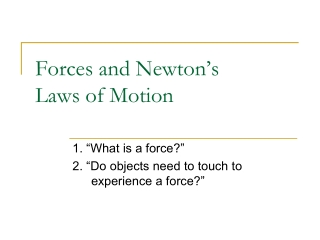DownloadDownload PresentationForces and Newton’s Laws of Motion

# Forces and Newton’s Laws of Motion

Télécharger la présentation## Forces and Newton’s Laws of Motion

- - - - - - - - - - - - - - - - - - - - - - - - - - - E N D - - - - - - - - - - - - - - - - - - - - - - - - - - -
##### Presentation Transcript

1. Forces and Newton’s Laws of Motion 1. “What is a force?” 2. “Do objects need to touch to experience a force?”

2. Forces and Newton’s Laws of Motion • Forces and Diagrams

3. What We Want to Know • What is force, and what types of forces are there? • What is the difference between mass and weight? • What are the advantages of drawing free-body diagrams when analyzing forces?

4. A Little History… Aristotle a famous Greek philosopher around 400 BC Natural Motion… objects seek their natural resting places Not caused by force Violent Motion… Result of forces that push or pull an object External cause… moving against nature No force… No motion Do you agree?

5. A Little History… Galileo the foremost scientist of the late-Renaissance Asked how things moved rather than why Demolished the notion that force is necessary to keep an object moving Only when friction is present is force required to keep objects moving Without frictional force, an object’s motion will continue Open the door for Isaac Newton and his Laws of Motion

6. Describe the ball • The ball’s motion will not change on its own. • Something must cause an objects motion to change.

7. Force • Force: a push or pull on an object which may change an objects state of rest or motion. • Forces cause a change in an object’s speed and/or direction. • Forces cause acceleration. • Forces are measured in Newtons (N). • Forces only exist as a result of objects interacting. • If their interaction stops, then there is no force.

8. Force • The forces that result from the interactions can be generalized as either contact forces or field forces.

9. Contact Forces • Contact Forces: forces resulting from actual physical contact between objects.

10. Field Forces • Field Forces: forces that are exerted from an interaction between objects, despite not being in physical contact with one another.

11. Contact Forces Applied Forces Tension Forces Spring Forces Frictional Forces Normal Force Field Forces Gravitational Forces Magnetic Forces Electrical Forces Examples

12. Weight vs. Mass • Weight is the force of gravity acting on an object. • The value of weight varies depending on where you are. ie. Earth, the Moon, another planet, etc. • Weight = mass x gravity (W = mg) • Mass is the amount of matter in an object. • The value of an objects mass is the same everywhere and does not change on location.

13. Normal Force • Normal Force: Results from contact with a surface. • The force that acts perpendicular to the surface.

14. Force (F) q Vertical Component Horizontal Component Vectors • Forces are vectors • Have a magnitude and direction. • Can be added or subtracted to form a resultant. • Can be resolved into components. • Force and free-body diagrams can help analyze forces at work.

15. Force and Free-body Diagrams • Force diagrams: shows all interacting objects and forces. • Free-body diagrams: help analyze situations by isolating a single object and the forces that are acting on that object.

16. Example Diagram

17. Practice • Draw a free-body diagram for: • A book sitting on a table. • A person starts a cart rolling down a ramp. • The cart rolling down a ramp. • A box at rest on a ramp. • A person pulling a sled across a frozen lake. • A bus being pushed over rocky terrain.

18. Objectives • What is force, and what types of forces are there? • What is the difference between mass and weight? • What are the advantages of drawing free-body diagrams when analyzing forces?

19. Exit Ticket • Reflect on your answers to “What is a force?” and “Do objects need to touch to experience a force?” • Are your answers different now than they were before? • Why did you think that way?